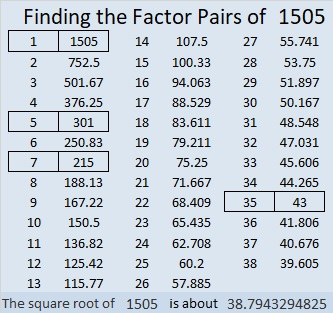# 1505 and Level 4

### Today’s Puzzle:

If you’ve never done a level 4 puzzle before, this one is a great choice. It has fewer tricky clues than usual. Start with a row or column that has two clues. Use logic and multiplication facts to figure out where to put the factors 1 to 10 in both the first column and the top row so that the given clues are the products of those factors. Have fun!### Factors of 1505:

• 1505 is a composite number.
• Prime factorization: 1505 = 5 × 7 × 43.
• 1505 has no exponents greater than 1 in its prime factorization, so √1505 cannot be simplified.
• The exponents in the prime factorization are 1, 1, and 1. Adding one to each exponent and multiplying we get (1 + 1)(1 + 1)(1 + 1) = 2 × 2 × 2 = 8. Therefore 1505 has exactly 8 factors.
• The factors of 1505 are outlined with their factor pair partners in the graphic below.### More about the Number 1505:

1505 has four different factor pairs. The numbers in each factor pair add up to an even number, so 1505 is the difference of two squares in four different ways. Here’s the complete list of those ways:
753² – 752² = 1505
153² – 148² = 1505
111² – 104² = 1505
39² – 4² = 1505